Foxtable(狐表)用户栏目专家坐堂 → 导入数据

共有316人关注过本帖树形打印复制链接

# 主题：导入数据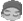fox-
1楼 | 信息 | 搜索 | 邮箱 | 主页 | UC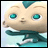加好友发短信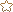老师您好，我想一下这个代码Dim dlg As New OpenFileDialogdlg.Filter = "Excel文件|*.xls;*.xlsx"If dlg.ShowDialog =DialogResult.OK Then       Dim App As New MSExcel.Application    try        Dim Wb As MSExcel.WorkBook = App.WorkBooks.Open(dlg.FileName)        Dim Ws As MSExcel.WorkSheet = Wb.WorkSheets(1)        Dim Rg As MSExcel.Range = Ws.UsedRange        Dim ary = rg.value        Dim dic As new Dictionary(of Integer, List(of object))        For Each s As object In ws.Shapes            Dim rng = s.TopLeftCell            If dic.ContainsKey(rng.Row) = False Then                Dim ls As new List(Of object)                ls.add(s)                dic.Add(rng.Row, ls)            Else                dic(rng.Row).add(s)            End If        Next        For n As Integer = 2 To rg.Rows.Count            Dim ro As Row = Tables("dd").AddNew            For i As Integer = 0 To Tables("dd").Cols.Count - 1                ro(i) = ary(n,i+1)            Next            If dic.ContainsKey(n) Then                Dim ls = dic(n)                Dim line As new List(Of String)                For j As Integer = 0 To ls.count-1                    Dim name = ary(n, 7) & "_" & j & ".jpg"                    ls(j).copy                    ClipBoard.GetImage.save(projectPath & "attachments/" & name)                    line.add(name)                Next                ro.DataRow.lines("照片") = line            End If        Next        MessageBox.Show("导入成功!","恭喜!")    catch ex As exception        msgbox(ex.message)        MessageBox.Show("导入失败!","恭喜!")    finally        app.quit    End tryEnd If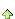fox-
2楼 | 信息 | 搜索 | 邮箱 | 主页 | UC加好友发短信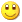Post By：2021/9/29 17:57:00 [只看该作者]

 Dim Book As New XLS.Book("c:\test\订单.xls")Dim Sheet As XLS.Sheet = Book.Sheets(0)Tables("订单").StopRedraw()'注意以下数组中列名称的顺序,必须和Excel表中的列顺序一致Dim nms() As String = {"编号","产品","客户","雇员","单价","折扣","数量","日期"}'注意下面的循环变量从1开始,而不是从0开始,因为Excel表的第一行是标题For n As Integer = 1 To Sheet.Rows.Count -1    Dim bh As String = sheet(n,0).Text    If DataTables("订单").Find("编号 = '" & bh & "'") Is Nothing Then '如果不存在同编号的订单        Dim r As Row = Tables("订单").AddNew()        For m As Integer = 0 To nms.Length - 1            r(nms(m)) = Sheet(n,m).Value 毕竟我只想要1楼的上传图片哪里，麻烦老师了，不懂3楼 | 信息 | 搜索 | 邮箱 | 主页 | UC加好友发短信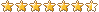Post By：2021/9/30 8:44:00 [只看该作者]

 只能使用vba处理，2楼的额用法用不了。如果只需要图片，把一楼代码去掉给表格赋值的代码即可fox-
4楼 | 信息 | 搜索 | 邮箱 | 主页 | UC加好友发短信Post By：2021/9/30 8:48:00 [只看该作者]

 If dic.ContainsKey(n) Then                Dim ls = dic(n)                Dim line As new List(Of String)                For j As Integer = 0 To ls.count-1                    Dim name = ary(n, 7) & "_" & j & ".jpg"                    ls(j).copy                    ClipBoard.GetImage.save(projectPath & "attachments/" & name)                    line.add(name)                Next 这一段吗，老师怎么把这个跟二楼结合，批量导入数据的同时，可以上传图片chnfo
5楼 | 信息 | 搜索 | 邮箱 | 主页 | UC加好友发短信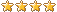Post By：2021/9/30 9:01:00 [只看该作者]

 这样可能会有问题的哟，因为图片可能来源于不同的路径，重名但实际不同的话，只能保留一个啊。除非加上ID6楼 | 信息 | 搜索 | 邮箱 | 主页 | UC加好友发短信Post By：2021/9/30 9:04:00 [只看该作者]

 没有办法结合，他们是完全不同的组件和用法。如果要在1楼的代码里指定导入列，可以这样Dim dlg As New OpenFileDialogdlg.Filter = "Excel文件|*.xls;*.xlsx"If dlg.ShowDialog =DialogResult.OK Then        Dim App As New MSExcel.Application    try        Dim Wb As MSExcel.WorkBook = App.WorkBooks.Open(dlg.FileName)        Dim Ws As MSExcel.WorkSheet = Wb.WorkSheets(1)        Dim Rg As MSExcel.Range = Ws.UsedRange        Dim ary = rg.value        Dim dic As new Dictionary(of Integer, List(of object))        For Each s As object In ws.Shapes            Dim rng = s.TopLeftCell            If dic.ContainsKey(rng.Row) = False Then                Dim ls As new List(Of object)                ls.add(s)                dic.Add(rng.Row, ls)            Else                dic(rng.Row).add(s)            End If        Next        Dim nms() As String = {"编号","产品","客户","雇员","单价","折扣","数量","日期"}        Dim s1 As new List(of String)        s1.Addrange(nms)        Dim dict As new Dictionary(of String,Integer)        For m As Integer = 1 To rg.Cols.Count            If s1.Contains(ary(0,m)) Then                dict.add(ary(0,m),m)            End If        Next        For n As Integer = 2 To rg.Rows.Count            If DataTables("订单").Find("编号 = '" & ary(n,dict("编号")) & "'") Is Nothing Then                Dim ro As Row = Tables("订单").AddNew                For i As Integer = 0 To nms.length - 1                    ro(i) = ary(n,dict(nms(i)))                Next                If dic.ContainsKey(n) Then                    Dim ls = dic(n)                    Dim line As new List(Of String)                    For j As Integer = 0 To ls.count-1                        Dim name = ary(n, 7) & "_" & j & ".jpg"                        ls(j).copy                        ClipBoard.GetImage.save(projectPath & "attachments/" & name)                        line.add(name)                    Next                    ro.DataRow.lines("照片") = line                End If            End If        Next        MessageBox.Show("导入成功!","恭喜!")    catch ex As exception        msgbox(ex.message)        MessageBox.Show("导入失败!","恭喜!")    finally        app.quit    End tryEnd Iffox-
7楼 | 信息 | 搜索 | 邮箱 | 主页 | UC加好友发短信Post By：2021/9/30 9:05:00 [只看该作者]

 老师我问的是二楼的代码他上传不到图片嘛，怎么结合4楼的代码可以上传11112
8楼 | 信息 | 搜索 | 邮箱 | 主页 | UC加好友发短信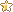Post By：2021/9/30 9:06:00 [只看该作者]

 谢谢老师9楼 | 信息 | 搜索 | 邮箱 | 主页 | UC加好友发短信Post By：2021/9/30 9:19:00 [只看该作者]

 二楼的代码这种用法无法获取图片，只能使用4楼的方法fox-
10楼 | 信息 | 搜索 | 邮箱 | 主页 | UC加好友发短信Post By：2021/9/30 9:26:00 [只看该作者]

 这个是range成员应该怎么改好呢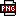此主题相关图片如下：1632965064(1).jpg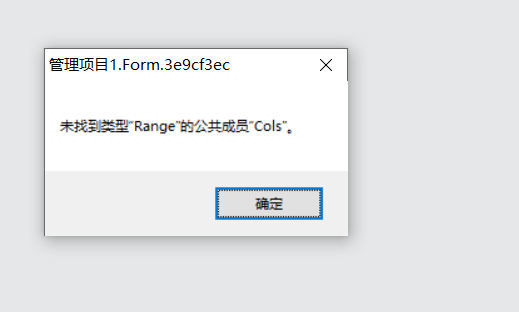总数 19 1 2 下一页